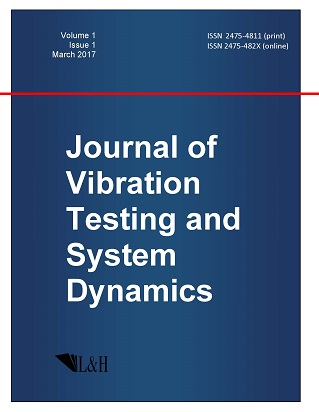ISSN: 2475-4811 (print)
ISSN: 2475-482X (online)
Journal of Vibration Testing and System Dynamics

C. Steve Suh (editor), Pawel Olejnik (editor),

Xianguo Tuo (editor)

Pawel Olejnik (editor)

Lodz University of Technology, Poland

Email: pawel.olejnik@p.lodz.pl

C. Steve Suh (editor)

Texas A&M University, USA

Email: ssuh@tamu.edu

Xiangguo Tuo (editor)

Sichuan University of Science and Engineering, China

Email: tuoxianguo@suse.edu.cn

On Preliminary Group Classification of the Isotropic Boltzmann Equation with a Source Term in a Problem of Homogeneous Relaxation by Using Extended Equivalence Group

Journal of Vibration Testing and System Dynamics 5(3) (2021) 259--267 | DOI:10.5890/JVTSD.2021.09.006

Yu.N.Grigoriev$^1$, S.V. Meleshko$^2$ , A. Suriyawichitseranee$^2$

$^1$ Institute of Computational Technology, Novosibirsk, 630090, Russia

$^2$ School of Mathematics, Institute of Science, Suranaree University of Technology, 30000, Thailand

Abstract

The paper is devoted to preliminary group classification of the spatially homogeneous and isotropic Boltzmann equation with a source term. In fact, the Fourier transform of the Boltzmann equation with respect to the molecular velocity variable is considered. The equivalence transformations considered earlier were extended by the change of time. This extends the set of source functions. The analysis of the extended equivalence group is given. Using optimal systems of finite-dimensional subalgebras of these extended equivalence set of transformations, preliminary classification of the source function is obtained.

References

1.   Chapman, S. and Cowling, T.G. (1994), \newblock { The mathematical theory of non-uniform gases}, \newblock Springer, New York.
2.   Boffi, V.C. and Spiga, G. (1982), \newblock Nonlinear diffusion of test particles in the presence of an external conservative force, \newblock { J. Phys. Fluids}, 25, 1987-1992.
3.   Ovsiannikov, L.V. (1978), \newblock { Group Analysis of Differential Equations}, \newblock Nauka, Moscow, 1978, \newblock {E}nglish translation, {A}mes, {W}.{F}., Ed., published by Academic Press, New York, 1982.
4.   Nonenmacher, T.F. (1984), \newblock Application of the similarity method to the nonlinear {B}oltzmann equation, \newblock { J. of Appl. Mathem. and Physics (ZAMP)}, 35(5), 680-691.
5.   Krook, M. and Wu, T.T. (1976), \newblock Formation of {M}axwellian tails, \newblock { Phys. Rev. Lett.}, 36(19), 1107-1109.
6.   Grigoriev, Y.N., Meleshko, S.V., and Suriyawichitseranee, A. (2014), \newblock On group classification of the spatially homogeneous and isotropic {B}oltzmann equation with sources {II}, \newblock { International Journal of Non-Linear Mechanics}, 61, 15-18.
7.   Bobylev, A.V. (1975), \newblock The method of {F}ourier transform in the theory of the {B}oltzmann equation for {M}axwell molecules, \newblock { Dokl. Akad. Nauk SSSR.}, 225, 1041-1044.
8.   Grigoriev, Y.N. and Meleshko, S.V. (1986), \newblock Investigation of invariant solutions of the {B}oltzmann kinetic equation and its models, \newblock Preprint of Institute of Theoretical and Applied Mechanics.
9.   Grigoriev, Y.N. and Meleshko, S.V. (1987), \newblock Group analysis of the integro-differential {B}oltzman equation, \newblock { Dokl. AS USSR}, 297(2), 323-327.
10.   Suriyawichitseranee, A., Grigoriev, Y.N., and Meleshko, S.V. (2015), \newblock Group analysis of the fourier transform of the spatially homogeneous and isotropic {B}oltzmann equation with a source term, \newblock { Communications in Nonlinear Science and Numerical Simulation}, 20, 719-730.
11.   Akhatov, I.S., Gazizov, R.K., and Ibragimov, N.H. (1991), \newblock Nonlocal symmetries. {H}euristic approach, \newblock { J. Math. Sci.}, 55(1), 1401-1450. \newblock Journal of Soviet Mathematics (in Russian).
12.   Ibragimov, N.H., Torrisi, M., and Valenti, A. (1991), \newblock Preliminary group classification of equations $v_{tt}=f(x,v_{x})v_{xx}+g(x,v_{x})$, \newblock { Journal of Mathematical Physics}, 32, 2988-2995.
13.   {Dos Santos Cardoso-Bihlo}, E., Bihlo, A., and Popovych, R.O. (2011), \newblock Enhanced preliminary group classification of a class of generalized diffusion equations, \newblock { Commun. Nonlinear Sci. Numer. Simul.}, 16, 3622-3638.
14.   Long, F.S., Karnbanjong, A., Suriyawichitseranee, A., Grigoriev, Y.N., and Meleshko, S.V. (2017), \newblock Application of a {L}ie group admitted by a homogeneous equation for group classification of a corresponding inhomogeneous equation, \newblock { Commun. Nonlinear Sci. Numer. Simulat.}, 48, 350-360.
15.   Bobylev, A.V. (1975), \newblock On exact solutions of the {B}oltzmann equation, \newblock { Dokl. AS USSR}, 225(6), 1296-1299.
16.   Spiga, G. (1984), \newblock A generalized bkw solution of the nonlinear {B}oltzmann equation with removal, \newblock { Physics of Fluids}, 27(11), 2599-2600.
17.   Santos, A. and Brey, J.J. (1985), \newblock Comments on `a generalized {BKW} solution of the nonlinear {B}oltzmann equation with removal, \newblock { Physics of Fluids}, 29(5), 1750.
18.   Grigoriev, Y.N., Meleshko, S.V., and Suriyawichitseranee, A. (2018), \newblock Exact solutions of the {B}oltzmann equation with a source, \newblock { Journal of Applied Mechanics and Technical Physics}.
19.   Meleshko, S.V. (2005), \newblock { Methods for Constructing Exact Solutions of Partial Differential Equations}, \newblock Mathematical and Analytical Techniques with Applications to Engineering. Springer, New York.
20.   Grigoriev, Y.N., Ibragimov, N.H., Kovalev, V.F., and Meleshko, S.V. (2010), \newblock { Symmetries of integro-differential equations and their applications in mechanics and plasma physics}, \newblock Lecture Notes in Physics, Vol. 806. Springer, Berlin / Heidelberg.
21.   Lie, S. (1880), \newblock Theorie der transformationsgruppen {I}, \newblock { Math. Ann.}, 16, 441-528.
22.   Ovsiannikov, L.V. (1993), \newblock On optimal system of subalgebras, \newblock { Dokl. RAS}, 333(6), 702-704.